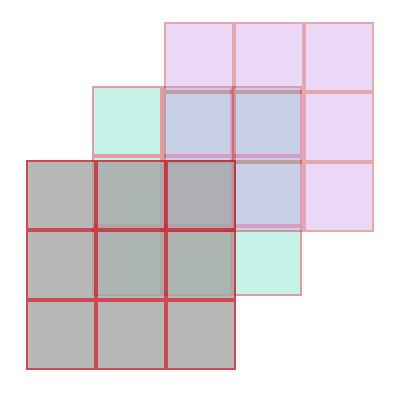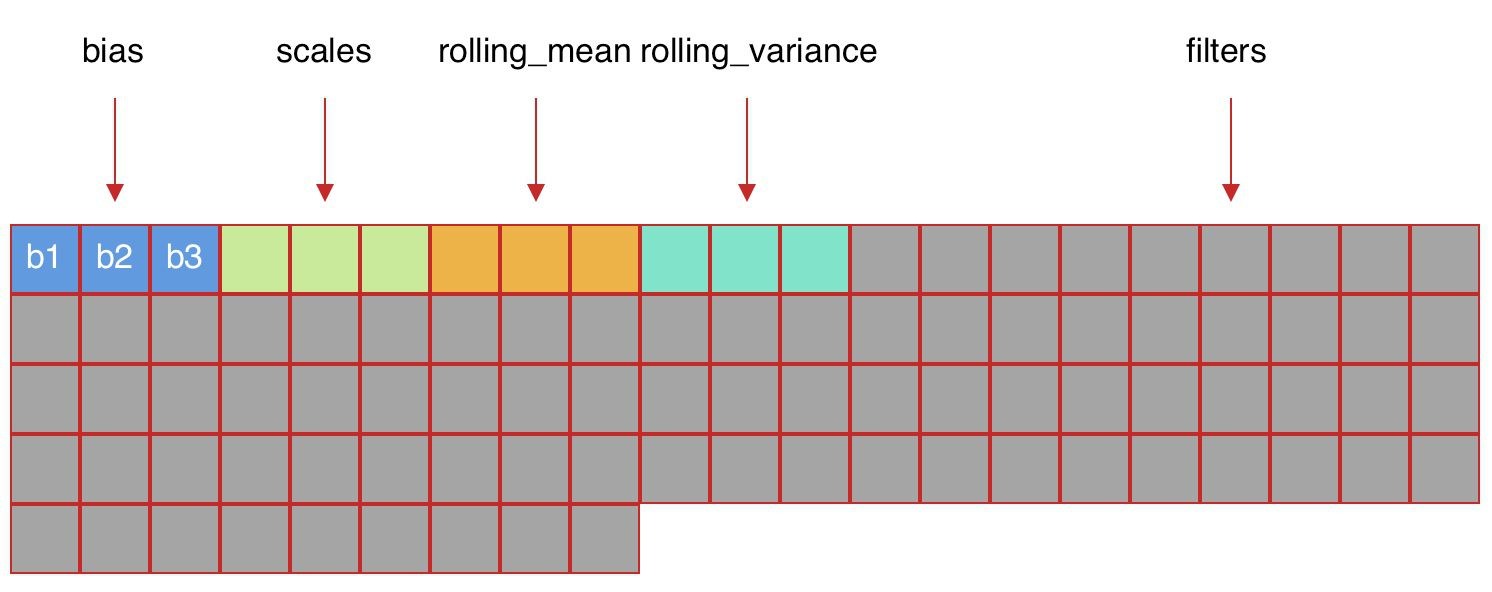RY 's Blog

The Weights of the YOLO Neural Network

2019-05-12

First, see this C library function:

Parameters

• ptr − Pointer to a block of memory with a size of at least (sizecount) bytes, converted to a void.

• size − This is the size in bytes of each element to be read.

• count − This is the number of elements, each one with a size of size bytes.

• stream − This is the pointer to a FILE object that specifies an input stream.

Then, in the load_weights_upto function in parser.c, it begins to load weights for layers from xxx.weights.

Convolutional Layer

In load_convolutional_weights function, it loads values of biases for filters. One bias for one filter.

l.n is the number of filter in this layer.

Assign the values to scales, rolling_mean and rooling_variance

And load weights for filters in this layer. The default value of l.groups is 1.

l.c is input channel. The number of input channel equal to the number of channel of a filter in this layer. Thus, l.size is the size of a filter.

For a 3x3x3 filter (size=3, channel=3), it has 3x3x3=27 parameters as followIf a convolutional layer has 3 such filters, it will have 3 values for bias, 3x3x3x3 .Its weight layout in memory is like this:Write Weights

save_weights_upto and save_convolutional_weightsfunctions show how to save weights into a xxx.weights file. It’s just a reverse process.

Parameters

• ptr
Pointer to the array of elements to be written, converted to a const void*.
• size
Size in bytes of each element to be written.
size_t is an unsigned integral type.
• count
Number of elements, each one with a size of size bytes.
size_t is an unsigned integral type.
• stream
Pointer to a FILE object that specifies an output stream.
Tags: YOLO